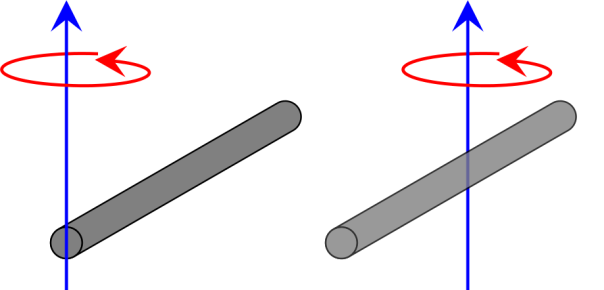# Rotational Kinetic Energy and Moment of Intertia

The kinetic energy of an object moving in a straight line is easy to understand:$E_\textrm{k} = \frac{1}{2}mv^2$

where$E_\textrm{k}$ is kinetic energy,$m$ is the mass of the object and$v$ is the object’s velocity.* If the mass of the object doubles, the kinetic energy doubles; and if the object’s velocity double the kinetic energy is quadrupled.

Kinetic energy is the energy that an object has due to the fact that it is moving, and therefore an object that is rotating must have kinetic energy. But if it’s rotating, staying in one place, then its velocity is zero and thus we can’t use the equation above.

The kinetic energy of a rotating object is given by:$E_\textrm{k} = \frac{1}{2}I\omega^2$

where$E_\textrm{k}$ is the rotational kinetic energy,$\omega$ is the angular velocity (i.e. the angle turned through divided by the time taken, measured in degrees per second or radians per second). The complicated part of the equation is$I$, which is the moment of inertia.

The moment of inertia can be thought of as the rotational equivalent of mass; the degree to which an object resists a change in its (rotational) motion. The moment of inertia depends on the shape of the object and the way it rotates. For example: the moment of inertia of a rod rotating about its centre, and a rod rotating about its end, are very different.The moment of inertia of the rod on the left, rotating around its end is$I = \frac{ml^2}{3}$, where$m$ is the mass of the rod and$l$ is its length. The moment of inertia of the rod on the right that rotates around its centre is$I = \frac{ml^2}{12}$ and thus if the rods have the same lengths and masses, and rotate at the same rate, the kinetic energy of the rod on the left will be four times greater.